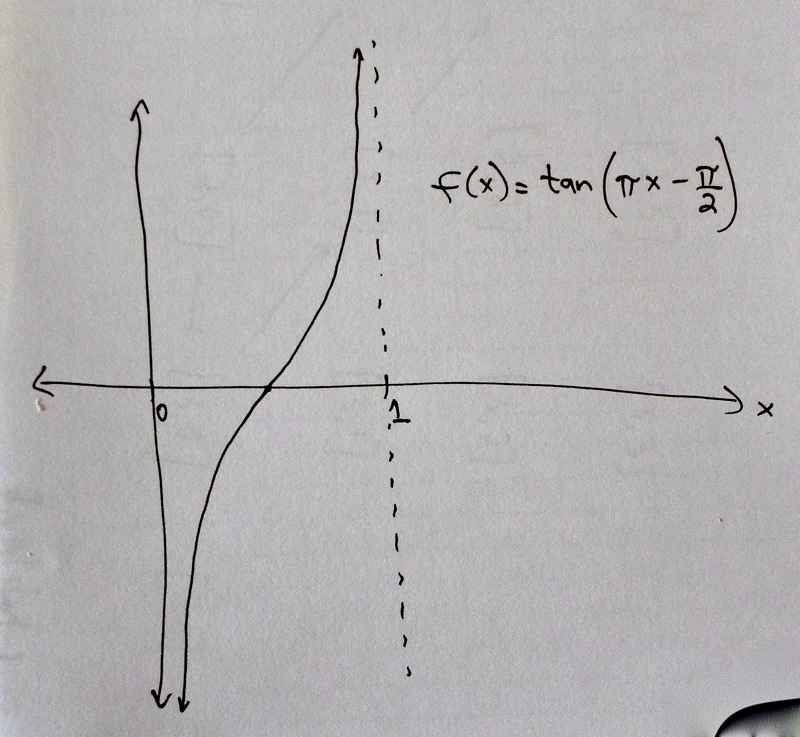# Short

Our goal is to get a rough idea of why $$|\mathbb{R}| = |(0,1)|.$$

To show that the sets $$\mathbb{R}$$ and $$(0,1)$$ are the same size, we would need to find a one-to-one correspondence between the elements of $$\mathbb{R}$$ and the elements of $$(0,1)$$.

We describe one-to-one correspondences using some assignment rule or pattern. When we did this with the sets $$\mathbb{N}$$ and $$2\mathbb{N}$$ in Basics of Set Theory, the pattern was: send every element of $$\mathbb{N}$$ to its double in $$2\mathbb{N}$$. This was a concise way of describing the assignment rule.

Mathematicians call this type of assignment rule a function. In the earlier example, we could write the function between $$\mathbb{N}$$ and $$2\mathbb{N}$$ as $$f(x) = 2x$$. In other words, if you input $$x$$ into the function, it outputs $$2$$ times $$x$$.

Some, but not all, one-to-one correspondences can be written concisely as functions. Not every function between two sets is a one-to-one correspondence though! We still have to check that it sends exactly one element to exactly one element, and doesn't miss any.

In the case of $$\mathbb{R}$$ and $$(0,1)$$, there is a nice function that we can use, and it is in fact a one-to-one correspondence. In the In-Depth explanation we'll go into more detail about why this function is a one-to-one correspondence between the set $$(0,1)$$ and $$\mathbb{R}$$. In this section we’ll just tell you what the function is and show you a picture.

The function actually starts with an element of the set $$(0,1)$$, and assigns an element of $$\mathbb{R}$$. So let $$x$$ be an element of $$(0,1)$$. Then the function assigns $$x$$ to $$f(x) = \tan(\pi x - \pi/2)$$.

The phrase "tan" means this is a form of the tangent function, from trigonometry. (The reason we wanted to leave $$t=0$$ and $$t=1$$ out of our set corresponding to breathed infinity, is because this function $$f$$ breaks if we try to plug in $$0$$ or $$1$$.) Here is a graph of this function.Top